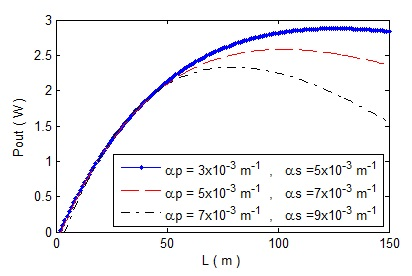# Theoretical analysis of Yb3+-doped double-clad fiber lasers using a new analytical method

\$59

## Description

The approximate analytical solutions to the threshold pump power and output laser power of Yb3+-doped double-clad fiber lasers have been deduced based on the rate equations with the scattering losses of pump and laser signal. In the rate equations, the relation between the attenuation of the pump light and the population density on the upper level is considered. The characteristics of Yb3+-doped double-clad fiber lasers have been investigated.

## Introduction

In recent years, rare earth-doped fiber lasers have drawn considerable attentions and made large progress experimentally [1–4] and theoretically [5–14]. Among these fiber lasers double-clad fiber lasers (DCFLs) have been actively studied. It is because of their characteristics including high output power, high conversion efficiency, easy heat extraction, excellent beam quality, large ration of surface area to volume, etc. Based on the rate equations for linear-cavity DCFL, Kelson and Hardy [7,10] have deduced the approximate analytic expressions of output power, lasing threshold, slope efficiency, the optimal fiber length and reflectivity of output mirror without the scattering loss. Xiao et al.  have deduced the approximate analytic solutions with scattering loss under the condition of the laser being strongly pumped, and shown three different pump modes. Kim et al.  and Peng et al.  have respectively investigated the DCFLs by numerical simulations based on the rate equations. However, one usually needs to guess the set of initial values in the numerical simulations, the results will be unstable if the initial values are not properly chosen. Luo et al.  and Liao et al.  have deduced the approximate analytic solution to the Yb3+- doped DCFL using different algorithms, respectively. However, in these two works, the attenuation coefficient of the pump light along the fiber has been approximately regarded as a constant, that is the attenuation of the pump light have no relation with the change of the population density on the upper level. In this paper, it is considered that the attenuation coefficient of the pump light depends on the population density of the upper level. The characteristics of the DCFL have been investigated based on the rate equations with

## Conclusion

In this paper, the threshold pump power and output laser power of the DCFL have been investigated based on the rate equations. It is found that the bigger value of R2 results of more laser photon density in the cavity and lower threshold pump power, but leads to lower output laser power. There is a minimum fiber length (Lmin) to emit output laser signal. The laser with bigger loss coefficients of pump and laser has a shorter Lopt. And the laser with longer fiber length and bigger dopant concentration has a smaller value of Ropt.

## Result :Analysis of pointillism art algorithm

MATLAB code for Hovering Hummingbirds Image Analysis

## Reviews

There are no reviews yet.